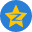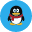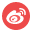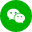### 图像隐写之使用PHP隐藏图像中的文本发布于 2018-02-07 17:43

# 相关的原理

11001100 10010001 00101011

00011000 11110000 11111110

11100010 00100101 01010101

11111101 00001010 01000011

11001100 10010001 00101011

00011000 11110000 11111111

11100010 00100101 01010100

11111101 00001010 01000011

# 用PHP实现

encrypt.php

decrypt.php

functions.php

1.jpg

encrypt.php

include('functions.php');

\$message_to_hide = 'hello';

\$binary_message = toBin(\$message_to_hide);

\$message_length = strlen(\$binary_message);

\$src = '1.jpg';

\$im = imagecreatefromjpeg(\$src);

for(\$x=0;\$x<\$message_length;\$x++){

\$y = \$x;

\$rgb = imagecolorat(\$im,\$x,\$y);

\$r = (\$rgb >>16) & 0xFF;

\$g = (\$rgb >>8) & 0xFF;

\$b = \$rgb & 0xFF;

\$newR = \$r;

\$newG = \$g;

\$newB = toBin(\$b);

\$newB[strlen(\$newB)-1] = \$binary_message[\$x];

\$newB = toString(\$newB);

\$new_color = imagecolorallocate(\$im,\$newR,\$newG,\$newB);

imagesetpixel(\$im,\$x,\$y,\$new_color);

}

echo \$x;

imagepng(\$im,'simple.png');

imagedestroy(\$im);

?>

decrypt.php

include('functions.php');

\$src = 'simple.png';

\$im = imagecreatefrompng(\$src);

\$real_message = '';

for(\$x=0;\$x<40;\$x++){

\$y = \$x;

\$rgb = imagecolorat(\$im,\$x,\$y);

\$r = (\$rgb >>16) & 0xFF;

\$g = (\$rgb >>8) & 0xFF;

\$b = \$rgb & 0xFF;

\$blue = toBin(\$b);

\$real_message .= \$blue[strlen(\$blue)-1];

}

\$real_message = toString(\$real_message);

echo \$real_message;

die;

?>

functions.php

function toBin(\$str){

\$str = (string)\$str;

\$l = strlen(\$str);

\$result = '';

while(\$l--){

\$result = str_pad(decbin(ord(\$str[\$l])),8,"0",STR_PAD_LEFT).\$result;

}

return \$result;

}

function toString(\$binary){

return pack('H*',base_convert(\$binary,2,16));

}

?>最新评论 (0)